Search by Topic

Resources tagged with Working systematically similar to Shady Symmetry:

Filter by: Content type:
Age range:
Challenge level:

There are 127 results

Broad Topics > Using, Applying and Reasoning about Mathematics > Working systematicallyAge 11 to 14 Challenge Level:

How many different symmetrical shapes can you make by shading triangles or squares?Cuboids

Age 11 to 14 Challenge Level:

Find a cuboid (with edges of integer values) that has a surface area of exactly 100 square units. Is there more than one? Can you find them all?Triangles to Tetrahedra

Age 11 to 14 Challenge Level:

Imagine you have an unlimited number of four types of triangle. How many different tetrahedra can you make?Summing Consecutive Numbers

Age 11 to 14 Challenge Level:

15 = 7 + 8 and 10 = 1 + 2 + 3 + 4. Can you say which numbers can be expressed as the sum of two or more consecutive integers?Isosceles Triangles

Age 11 to 14 Challenge Level:

Draw some isosceles triangles with an area of $9$cm$^2$ and a vertex at (20,20). If all the vertices must have whole number coordinates, how many is it possible to draw?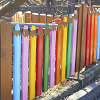Fence It

Age 11 to 14 Challenge Level:

If you have only 40 metres of fencing available, what is the maximum area of land you can fence off?Where Can We Visit?

Age 11 to 14 Challenge Level:

Charlie and Abi put a counter on 42. They wondered if they could visit all the other numbers on their 1-100 board, moving the counter using just these two operations: x2 and -5. What do you think?Consecutive Negative Numbers

Age 11 to 14 Challenge Level:

Do you notice anything about the solutions when you add and/or subtract consecutive negative numbers?Squares in Rectangles

Age 11 to 14 Challenge Level:

A 2 by 3 rectangle contains 8 squares and a 3 by 4 rectangle contains 20 squares. What size rectangle(s) contain(s) exactly 100 squares? Can you find them all?More Magic Potting Sheds

Age 11 to 14 Challenge Level:

The number of plants in Mr McGregor's magic potting shed increases overnight. He'd like to put the same number of plants in each of his gardens, planting one garden each day. How can he do it?Tetrahedra Tester

Age 11 to 14 Challenge Level:

An irregular tetrahedron is composed of four different triangles. Can such a tetrahedron be constructed where the side lengths are 4, 5, 6, 7, 8 and 9 units of length?Maths Trails

Age 7 to 14

The NRICH team are always looking for new ways to engage teachers and pupils in problem solving. Here we explain the thinking behind maths trails.Window Frames

Age 5 to 14 Challenge Level:

This task encourages you to investigate the number of edging pieces and panes in different sized windows.Special Numbers

Age 11 to 14 Challenge Level:

My two digit number is special because adding the sum of its digits to the product of its digits gives me my original number. What could my number be?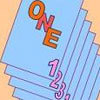One Out One Under

Age 14 to 16 Challenge Level:

Imagine a stack of numbered cards with one on top. Discard the top, put the next card to the bottom and repeat continuously. Can you predict the last card?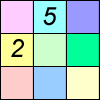Difference Sudoku

Age 14 to 16 Challenge Level:

Use the differences to find the solution to this Sudoku.Two and Two

Age 11 to 14 Challenge Level:

How many solutions can you find to this sum? Each of the different letters stands for a different number.Problem Solving, Using and Applying and Functional Mathematics

Age 5 to 18 Challenge Level:

Problem solving is at the heart of the NRICH site. All the problems give learners opportunities to learn, develop or use mathematical concepts and skills. Read here for more information.Sticky Numbers

Age 11 to 14 Challenge Level:

Can you arrange the numbers 1 to 17 in a row so that each adjacent pair adds up to a square number?Games Related to Nim

Age 5 to 16

This article for teachers describes several games, found on the site, all of which have a related structure that can be used to develop the skills of strategic planning.Multiply the Addition Square

Age 11 to 14 Challenge Level:

If you take a three by three square on a 1-10 addition square and multiply the diagonally opposite numbers together, what is the difference between these products. Why?Age 7 to 14 Challenge Level:

I added together some of my neighbours' house numbers. Can you explain the patterns I noticed?9 Weights

Age 11 to 14 Challenge Level:

You have been given nine weights, one of which is slightly heavier than the rest. Can you work out which weight is heavier in just two weighings of the balance?Instant Insanity

Age 11 to 18 Challenge Level:

Given the nets of 4 cubes with the faces coloured in 4 colours, build a tower so that on each vertical wall no colour is repeated, that is all 4 colours appear.Product Sudoku

Age 11 to 14 Challenge Level:

The clues for this Sudoku are the product of the numbers in adjacent squares.M, M and M

Age 11 to 14 Challenge Level:

If you are given the mean, median and mode of five positive whole numbers, can you find the numbers?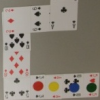Sociable Cards

Age 11 to 14 Challenge Level:

Move your counters through this snake of cards and see how far you can go. Are you surprised by where you end up?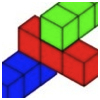Building with Longer Rods

Age 7 to 14 Challenge Level:

A challenging activity focusing on finding all possible ways of stacking rods.Difference Dynamics

Age 14 to 18 Challenge Level:

Take three whole numbers. The differences between them give you three new numbers. Find the differences between the new numbers and keep repeating this. What happens?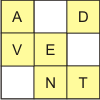Age 11 to 14 Challenge Level:

Rather than using the numbers 1-9, this sudoku uses the nine different letters used to make the words "Advent Calendar".Weights

Age 11 to 14 Challenge Level:

Different combinations of the weights available allow you to make different totals. Which totals can you make?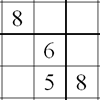Twin Line-swapping Sudoku

Age 14 to 16 Challenge Level:

A pair of Sudoku puzzles that together lead to a complete solution.The Great Weights Puzzle

Age 14 to 16 Challenge Level:

You have twelve weights, one of which is different from the rest. Using just 3 weighings, can you identify which weight is the odd one out, and whether it is heavier or lighter than the rest?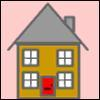Smith and Jones

Age 14 to 16 Challenge Level:

Mr Smith and Mr Jones are two maths teachers. By asking questions, the answers to which may be right or wrong, Mr Jones is able to find the number of the house Mr Smith lives in... Or not!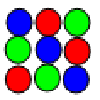Latin Squares

Age 11 to 18

A Latin square of order n is an array of n symbols in which each symbol occurs exactly once in each row and exactly once in each column.American Billions

Age 11 to 14 Challenge Level:

Play the divisibility game to create numbers in which the first two digits make a number divisible by 2, the first three digits make a number divisible by 3...LOGO Challenge - Sequences and Pentagrams

Age 11 to 18 Challenge Level:

Explore this how this program produces the sequences it does. What are you controlling when you change the values of the variables?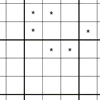Age 11 to 14 Challenge Level:

You need to find the values of the stars before you can apply normal Sudoku rules.Magic Caterpillars

Age 14 to 18 Challenge Level:

Label the joints and legs of these graph theory caterpillars so that the vertex sums are all equal.Teddy Town

Age 5 to 14 Challenge Level:

There are nine teddies in Teddy Town - three red, three blue and three yellow. There are also nine houses, three of each colour. Can you put them on the map of Teddy Town according to the rules?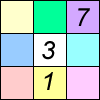Age 11 to 16 Challenge Level:

Four small numbers give the clue to the contents of the four surrounding cells.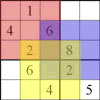Seasonal Twin Sudokus

Age 11 to 16 Challenge Level:

This pair of linked Sudokus matches letters with numbers and hides a seasonal greeting. Can you find it?Pole Star Sudoku 2

Age 11 to 16 Challenge Level:

This Sudoku, based on differences. Using the one clue number can you find the solution?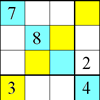Rectangle Outline Sudoku

Age 11 to 16 Challenge Level:

Each of the main diagonals of this sudoku must contain the numbers 1 to 9 and each rectangle width the numbers 1 to 4.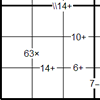Bochap Sudoku

Age 11 to 16 Challenge Level:

This Sudoku combines all four arithmetic operations.LCM Sudoku II

Age 11 to 18 Challenge Level:

You are given the Lowest Common Multiples of sets of digits. Find the digits and then solve the Sudoku.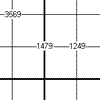Another Quadruple Clue Sudoku

Age 11 to 16 Challenge Level:

This is a variation of sudoku which contains a set of special clue-numbers. Each set of 4 small digits stands for the numbers in the four cells of the grid adjacent to this set.Star Product Sudoku

Age 11 to 16 Challenge Level:

The puzzle can be solved by finding the values of the unknown digits (all indicated by asterisks) in the squares of the $9\times9$ grid.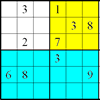Colour Islands Sudoku

Age 11 to 14 Challenge Level:

An extra constraint means this Sudoku requires you to think in diagonals as well as horizontal and vertical lines and boxes of nine.